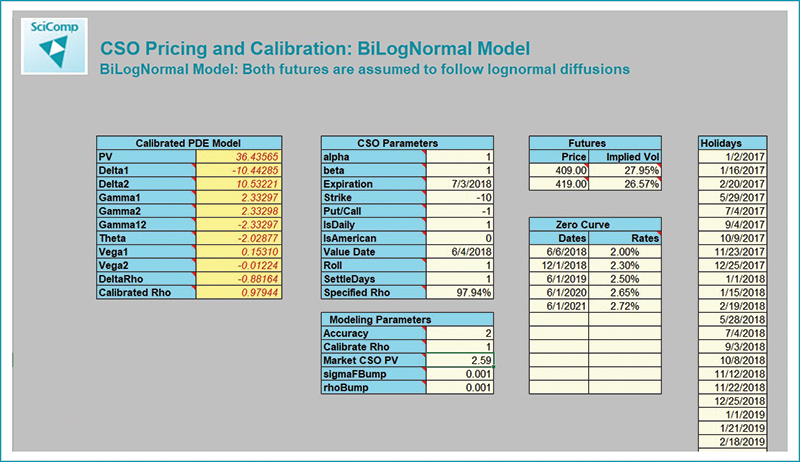Commodity Spread Option Pricing Model | SciComp Inc.

# Commodity Spread Option Pricing Model

SciComp’s Commodity Spread Option Pricing Models feature underlying futures dynamics based on either a lognormal model or the Gabillon futures model. The models price both intra-commodity spread options, e.g. Calendar Spread Options (CSOs) and inter-commodity spread options, e.g. Corn – Coffee Spread options. The models are particularly well suited for valuing structures in markets that may or may not have liquid quotes for spread options available for model calibration.

The lognormal Commodity Spread Option Pricing Model utilizes a two-factor lognormal diffusion model (BiLogNormal Spread Model) for pricing financial contracts based on an underlying spread between two futures contracts. The correlation of futures may calibrated to a single liquid spread option assuming constant volatilities, or alternatively, a local volatility model for each future may be calibrated to each futures volatility smile in addition to the correlation calibration.  If liquid spread option quotes are not available users may specify a correlation.  Similarly, the Gabillon based model calibrates a constant parameter Gabillon model to each underlying and then calibrates the correlation to liquid spread quotes or uses exogenously supplied correlations.

Like all SciComp ready-to-use solutions the Commodity Spread Option Pricing Models can be enhanced/modified to meet your particular modeling needs and is available as C/C++ source code, Windows/Linux executable, or an Excel spreadsheet and add-in.

### Lognormal Commodity Spread Option Pricing Model Output Parameters

• PV - Present value of the Spread option
• Delta1 - d(PV)/d(F1Spot)
• Delta2 - d(PV)/d(F2Spot)
• Gamma1 - d^2(PV)/d(F1Spot)^2
• Gamma2 - d^2(PV)/d(F2Spot)^2
• Gamma12 - d^2(PV)/(d(F1Spot)d(F2Spot))
• Theta - Change in PV when value date is advanced one day
• Vega1 - Change in PV when F1Vol is incremented
• Vega2 - Change in PV when F2Vol is incremented
• DeltaRho - Change in PV when correlation is incremented
• CalibratedRho – Switch to choose between calibrated or
specified correlation